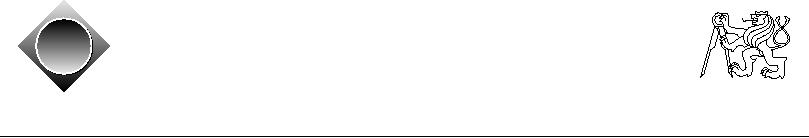Czech ACM Student Chapter
Czech Technical University in Prague
Charles University in Prague
Technical University of Ostrava
acm
ˇ a
Slovak University of Technology
Pavol Jozef Saf´rik University in Koˇice
s
cz
ˇ
University of Zilina
Masaryk University
Matej Bel University in Bansk´ Bystrica
a
University of West Bohemia
CTU Open Contest 2011
Collatz Conjecture
collatz.c, collatz.C, collatz.java, collatz.p
The Collatz Conjecture is an interesting phenomenon. Though its principle is very simple, it still
remains among unresolved problems in mathematics, even after many years of study. However,
the years of intensive research brought at least some results, which is a huge advantage of the
human race against the aliens, because they did not study the conjecture for so many years. We
Imagine a sequence defined recursively as follows: Start with any positive integer x0 (so-called
"starting value"). Then repeat the following:
· if xi is even, then xi+1 = xi/2 ("half ...")
· if xi is odd, then xi+1 = 3xi + 1 ("... or triple plus one")
The Collatz Conjecture says that every such sequence will eventually reach 1. It has still not
been proven until today but we already know for sure that this is true for every x0 < 258. (Never
tell this to aliens!)
In this problem, you are given two starting values and your task is to say after how many steps
their sequences "meet" for the first time (which means the first number that occurs in both
sequences) and at which number is it going to happen. For simplicity, we will assume that the
sequence does not continue once it has reached the number one. In reality, it would then turn
into 1, 4, 2, 1, 4, 2, 1, . . . , which quickly becomes boring.
Input Specification
The input contains several test cases. Each test case is described by a single line containing two
integer numbers A and B, 1 A, B 1 000 000.
The last test case is followed by a line containing two zeros.
Output Specification
For each test case, output the sentence "A needs SA steps, B needs SB steps, they meet
at C ", where SA and SB are the number of steps needed in both sequences to reach the same
number C . Follow the output format precisely.
Sample Input
78
27 30
00
Output for Sample Input
7 needs 13 steps, 8 needs 0 steps, they meet at 8
27 needs 95 steps, 30 needs 2 steps, they meet at 46